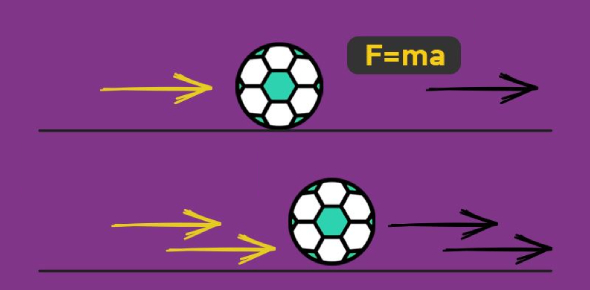Newton's Laws Of Motion Quiz Questions!

10 Questions | Total Attempts: 922Settings.

• 1.
A student wants to set up an experiment to determine the effect of a net force on an object’s acceleration. To do this, she should
• A.

Vary the force acting on the object and the mass of the object at the same time

• B.

Vary the mass of the object, but not the force acting on the object

• C.

Vary the force acting on the object, but not the object’s mass

• D.

Keep both the force acting on the object and the mass of the object constant as it rolls along a horizontal surface

• 2.
A 2 kg block is dropped from the roof of a tall building at the same time a 6 kg ball is thrown horizontally from the same height. Which statement best describes the motion of the block and the motion of the ball?
• A.

The 2 kg block hits the ground first because it has no horizontal velocity

• B.

The 6 kg ball hits the ground first because it is round

• C.

The 6 kg ball hits the ground first because it has more mass

• D.

The block and the ball hit the ground at the same time because they have the same vertical acceleration

• 3.
A student is holding a book that has a weight of 20N in her hand while sitting in a chair. She claims that the book must be attracting Earth with a force of 20 N. Her claim must be
• A.

False because books do not attract objects

• B.

False because Earth is much larger than the book

• C.

True because the book has more inertia than Earth

• D.

True due to Newton’s third law of motion

• 4.
Which diagram of a 5 kg mass resting on a table correctly represents the force (not mass!) of the table on the mass?
• A.

Image a is correct

• B.

Image b is correct

• C.

Image c is correct

• D.

Image d is correct

• 5.
A cart is rolling along a frictionless horizontal surface. Which of the following describes the motion of the cart as it continues to roll along the surface?
• A.

The cart will slow down as it runs out of forward force

• B.

The cart will continue to roll with constant speed

• C.

The cart will continue to roll with constant speed only if it is rolling downhill

• D.

The cart will slow down as it uses up its speed

• 6.
Which object has the most inertia?
• A.

A 0.001 kg bumblebee traveling at 2 m/s

• B.

A 0.1 kg baseball traveling at 20 m/s

• C.

A 5 kg bowling ball traveling at 3 m/s

• D.

A 10 kg tricycle at rest

• 7.
Two students have a tug-of-war on a smooth gym floor. One student has a mass of 70 kg and is wearing socks but no shoes. The other student has a mass of 60 kg and is wearing athletic shoes. The student most likely to win will be
• A.

The 60 kg student because he can pull harder than the 70 kg student

• B.

The 70 kg student because he can pull harder than the 60 kg student

• C.

The 60 kg student because he experiences greater frictional force with the floor

• D.

The 70 kg student because he experiences a greater frictional force with the floor

• 8.
A stone with an initial velocity of zero is dropped from a bridge above a river. After 3 s, the stone strikes the water below the bridge. Approximately how fast is the stone traveling when it strikes the water?
• A.

10 m/s

• B.

20 m/s

• C.

30 m/s

• D.

45 m/s

• 9.
If the sum of all forces acting on a moving object is zero, the object will
• A.

Continue moving with constant speed

• B.

Slow down and stop

• C.

Change the direction of its motion

• D.

Either speed up or slow down

• 10.
A soccer ball is kicked across a field. After it is no longer touching the player's foot, the ball continues to move because
• A.

No force is needed for the ball to keep moving at a constant speed

• B.

The force from the foot continues to push the ball forward, but eventually runs out

• C.

There is a force of motion that keeps the ball going

Related TopicsBack to top